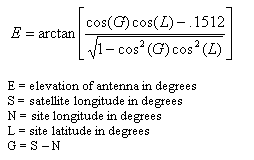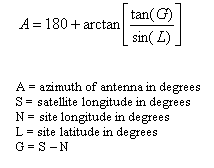Volume 3 No.2, Spring 1999 ISSN# 1523-9926

Computing Azimuth and Elevation Angles
with JavaScript

prepared by

 Jeffrey S. Beasley Joseph T. Page II jbeasley@nmsu.edu jopage@nmsu.edu Department of Engineering Technology New Mexico State University Department of Engineering Technology New Mexico State University

Abstract

This paper presents a method for calculating the "look angles" or "Az-El" (azimuth and elevation angles) for satellite earth stations using an on-line JavaScript program. The paper provides a brief overview of the equations required for performing the calculations and concludes with the source code for the JavaScript program.

Many communication links use satellites, parked in geostationary orbit (sometimes called geosynchronous) for transmitting information regionally and globally. Geostationary means that the satellite is located at a fixed point approximately 22,300 miles in altitude above the equator. At this altitude, the gravitational pulls of the earth, sun and the moon work together along with the centrifugal force caused by the satellites rotation around the earth to keep the satellite at a fixed location above the earth. Of course the satellite does drift (it moves in a figure eight pattern) and must be periodically repositioned using on-board power thrusters for maintaining the optimum location. But for us on earth, the satellite appears to be stationary. The satellite communication systems consist of the following:

• orbiting satellite

The uplink and downlink consist of an earth station (ground base station) which will typically be transmitting and receiving data, video and/or audio. Included in the earth station are items such as the exciter, the high power TWTA's - traveling wave tube amplifiers (also called HPA's - high power amplifiers), the receivers and a parabolic shaped reflector which is pointing at the satellites parked in geostationary orbit. The satellites require a payload of antennae, transponders (combination receive and transmit units) and attitude controls for maintaining the location in geostationary orbit. Orbital corrections (station keeping) on the satellite are made approximately every two to six weeks. The satellites are parked over the equator (latitude = 0) in geostationary orbit at a fixed longitude. The longitudinal position is referenced to earth at the sub-satellite point. The minimum spacing of the satellites is currently 2 degrees.

Geostationary satellite communications has many advantages over terrestrial microwave communications and low-orbit satellites. The geostationary satellite position is fixed with respect to earth. Secondly, the path to and from the satellite is always available except during certain weather conditions and solar disturbances. Additionally, the satellites transmission back to earth covers a very large area (footprint) which allows simultaneous transmission to many sites. Some disadvantages to geostationary satellites are propagation delays because of the distance to and from the satellite (approximately 44,600 miles round trip). Satellite transmitters require more power because of the increased distance (increased path loss) resulting in a significant increase in the transmitter costs. (It is difficult to fabricate high power amplifiers which operate at high frequencies). The last disadvantage is that there is a significant cost in maintaining a satellite parked in geostationary orbit.

The most common frequency bands used in satellite communications are C-band and Ku-band. Table 1 provides a list of frequencies commonly used in satellite communications for many different applications such as data, voice, entertainment, news, military, international broadcast and many other uses.

TABLE 1

C 5.9 - 6.4 3.7 - 4.2 500
X 7.9 - 8.4 7.25 - 7.75 500
Ku 14 - 14.5 11.7 - 12.2 500
Ka 27 - 30 17 - 20 Not fixed
30 - 31 20 - 21 Not Fixed

Table 1 does not list all of the satellite frequencies used in the United State but provides the major frequency bands currently in use. A good source for current satellites and their location and use in provided regularly in the WESTSAT publication .

## Azimuth and Elevation Calculations

The azimuth and elevation angles for our earth station antenna must be calculated so that the correct satellite can be seen. The azimuth is the horizontal pointing angle of the earth station antenna. The elevation is the angle we look up into the sky to see the satellite. To calculate the azimuth and elevation of a ground station antenna requires that the ground station latitude and longitude be known as well as the longitude of the satellite. The longitude of the satellite can be obtained from charts such as the WESTSAT. The latitude and longitude of the ground station can be obtained from U.S. Geological Survey maps of the region you are in. More advanced methods of locating the exact ground station are available through the use of the Global Positioning Satellite (GPS). Once the actual location is known the elevation angle of the earth station antenna can be calculated using equation 1.[Eq. 1]

Next the azimuth can be calculated using equation 2.[Eq. 2]

An example of how to use equations 1 and 2 is provided in Example 1.

Example 1:
Calculate the azimuth and elevation angles for an earth station (ground station) antenna at site latitude of 35 degrees north and a site longitude of 90 degrees west, aimed at geostationary satellite longitude of 83 degrees west.

Solution:## JavaScript Azimuth and Elevation Calculator

JavaScript is a general-purpose interpreted programming language, with rudimentary object-oriented abilities. JavaScript is very popular on the Internet for tasks that would normally require lengthy server-side programs. Its capabilities have been integrated into Netscape Navigator and other web browsers, allowing site authors to build relatively complex web pages with little prior programming knowledge. Its 'client-side' capabilities allows JavaScript to include executable content in web pages. 

Figure 1 shows the form for inputting latitude and longitude information for your earth station and satellite. The calculations presented are for North latitude and West longitude locations. This calculator does not keep track of significant figures, with the form output displaying only 5 digits. The RESET button is provided to clear the form of all input and calculations and allow the user to start anew. This code was tested on Netscape Communicator 4.0 and Internet Explorer 3.0.

 EARTH STATION NORTH LATITUDE: degrees EARTH STATION WEST LONGITUDE: degrees SATELLITE WEST LONGITUDE: degrees AZIMUTH: degrees ELEVATION: degrees

Figure 1

## JavaScript Code

This short script is embedded into a <FORM>/<TABLE> combination for an aesthetically pleasing and easy-to-read form. The JavaScript code begins and ends with the <SCRIPT>/</SCRIPT> tag combination. The code can be entered anywhere in the web page, but it must come before the <FORM> structure that utilizes it. The following is the text used in the embedded JavaScript code.

```<SCRIPT language="Javascript">

function calc(form, l, n, s) {  	// Function parameter names correspond to table variables

// VARIABLES USED IN CALCULATIONS

var azi;
var ele;
var g = (s - n);

var grad = (g / 57.29578);
var lrad = (l / 57.29578);
// var nrad = (n / 57.29578);
// var srad = (s / 57.29578);

// AZIMUTH CALCULATIONS

azi = (3.14159 -

form.azimuth.value = azi * 57.29578;

// ELEVATION CALCULATIONS
ele = Math.atan((a * b -.1512)/(Math.sqrt(1 - (a*a) * (b*b))));

form.elevation.value = ele * 57.29578;
}
</SCRIPT>

```

The following HTML code lists how the calculator table (shown in Figure 1) was created.

## <FORM>/<TABLE> Code

```<FORM>

<TABLE border=1>

<TR>
<TD><B>EARTH STATION NORTH LATITUDE:</B></TD>
<TD><INPUT TYPE=TEXT SIZE=8 name="l">degrees</TD>
</TR>

<TR>
<TD><B>EARTH STATION WEST LONGITUDE:</B></TD>
<TD><INPUT TYPE=TEXT SIZE=8 name="n"> degrees</TD>
</TR>

<TR>
<TD><B>SATELLITE WEST LONGITUDE:</B></TD>
<TD><INPUT TYPE=TEXT SIZE=8 name="s"> degrees</TD>
</TR>

<TR>
<TD align = center><INPUT TYPE="reset" value="RESET" ></TD>
<TD align = center><INPUT TYPE=button VALUE="CALCULATE"
onclick='calc(this.form,this.form.l.value,this.form.n.value,this.form.s.value)'></TD>
</TR>

<TR>
<TD align=center><B>AZIMUTH:</B></TD>
<TD><INPUT TYPE=TEXT SIZE=6 name="azimuth"> degrees</TD>
</TR>

<TR>
<TD align=center>
<B>ELEVATION:</B></TD>
<TD><INPUT TYPE=TEXT SIZE=6 name="elevation"> degrees</TD>
</TR>

</TABLE>
</FORM>
```

## Conclusion

This paper has presented a techniques for using JavaScript to calculate the azimuth and elevations angles for a satellite earth station. The routine is suitable for calculations in the Northern Hemisphere and Western longitudes. The JavaScript program can be easily modified to accommodate other geographical locations.

## For Additional Information on Satellite Communications see:

• Ha, Tri T., "Digital Satellite Communications, 2nd Edition", McGraw-Hill, Inc., 1990